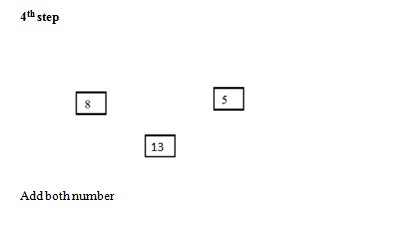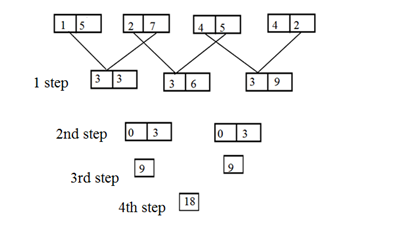# Crack SBI Clerk / IDBI Executive 2018 – Logical Reasoning (Miscellaneous) Day-36

Dear Readers, Reasoning Section play a vital role in Banking and all other competitive exams. This year 2018 was started with three wonderful opportunities; Most Awaited SBI Clerk 2018. To enrich your preparation here we have providing new series of Practice Questions on Reasoning Ability – Miscellaneous. Candidates those who are going to appear in Bank Exams 2018 can practice these questions daily and make your preparation effective.

[WpProQuiz 1587]

Click “Start Quiz” to attend these Questions and view Solutions

Directions (1-3): Read the information carefully and answer the given questions below

G is the father of D, who is the only aunt of B . I is the mother in law of E . H is the son in law of G who has only two children. E is the brother in law of F. B is the only grandchild of I. H has one Son. Neither E nor D is the spouse of H. Only three married couples are in the family

1) How is D related to I?

a) Daughter in law

b) Niece

c) Daughter

d) Mother

e) None of these

2) If M is the Cousin of B then, How is E related To M?

a) Aunt

b) Mother

c) Uncle

d) Father

e) None of these

3) How is F related to G?

a) Niece

b) Daughter

c) Daughter in law

d) Granddaughter

e) None of these

4) In which of the Following Expressions does the Expression S<R holds true and Y<S hold false?

a) R>Y<X≤S

b) R>X ≤Y≤S

c) R ≥Y>X ≥S

d) S<Y≥R ≥X

e) None of these

5) What Will come in place of question mark in the following expression D>X=M ? K≤ I? S, So that M≤ I  and K<S are Always true?

a) =, <

b) ≤ , =

c) > , <

d) ≥ , ≤

e) None of these

Directions (6-10): Study the following information carefully and answer the given questions.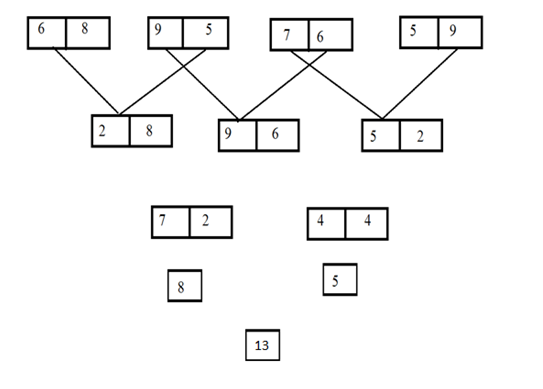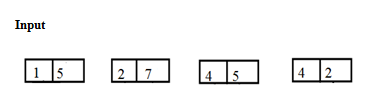6) What is the sum of second digit of largest number and first digit of smallest number in    step I?

a) 6

b) 18

c) 12

d) 16

e) 15

7) What is sum of numbers in step III?

a) 12

b) 14

c) 18

d) 21

e) 49

8) Which of the following represents the difference between the first value and the second value of Step III of the given input?

a) 2

b) 4

c) 5

d) 9

e) 0

9)  If the value “6” is added to the final output then what will be the resultant value?

a) 30

b) 24

c) 42

d) 81

e) 45

10)  If in the first step the first digit of every number is added and multiplied by 5 then which will be the resultant value?

a) 45

b) 65

c) 35

d) 40

e) 75

Directions (1-3)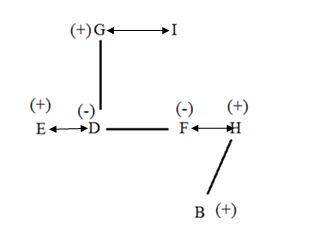4) Answer c) R ≥Y>X ≥S

D>X=M =K≤ I< S

Directions (6-10):

Logic

1st step4^2 + 4^2 = 16 + 16 = 32 = 3 + 2 = 5